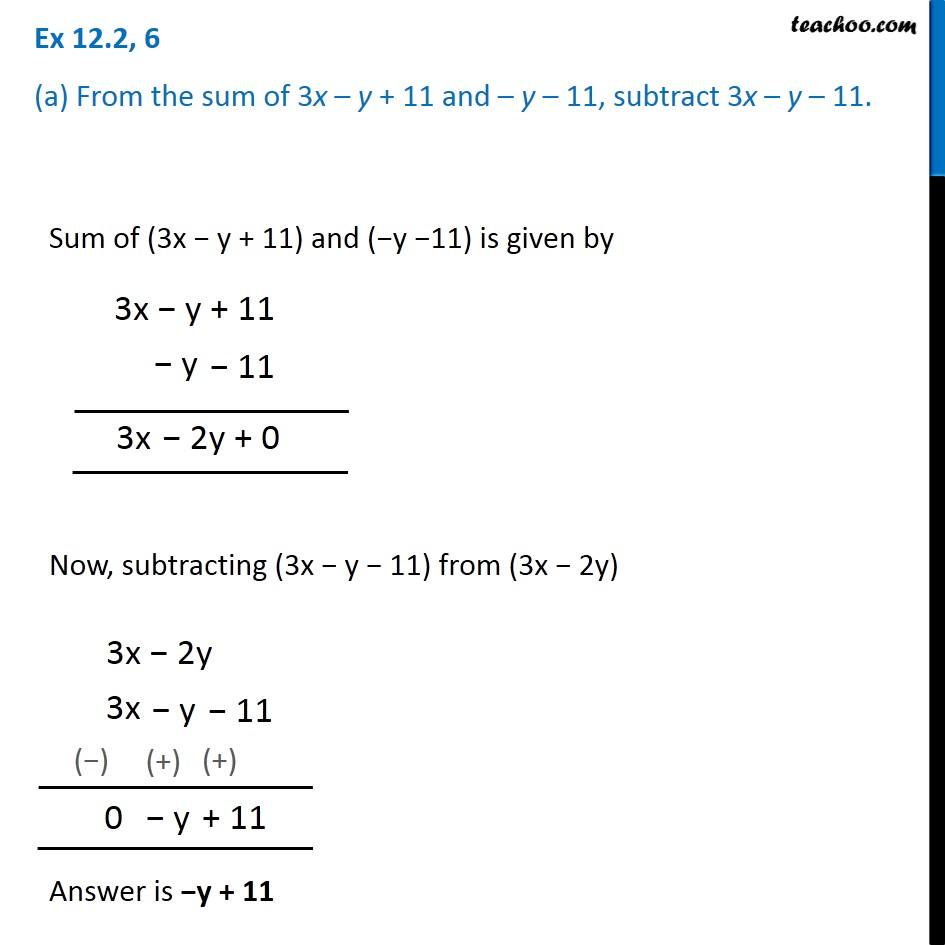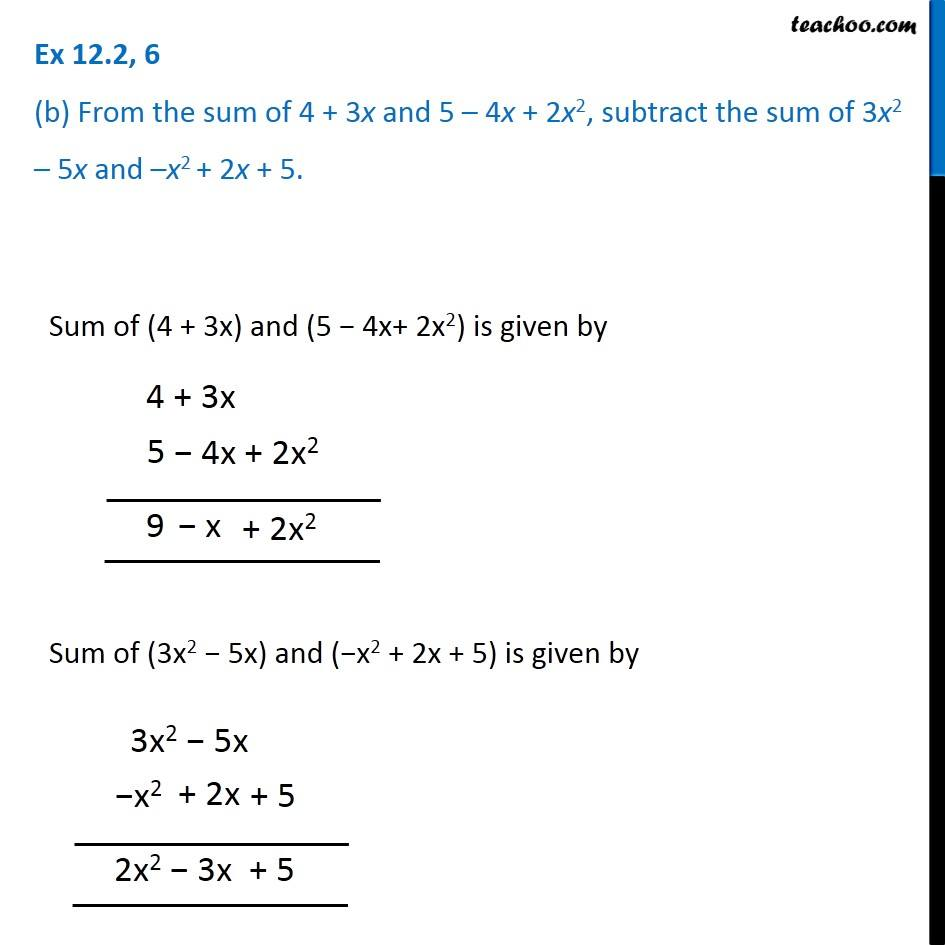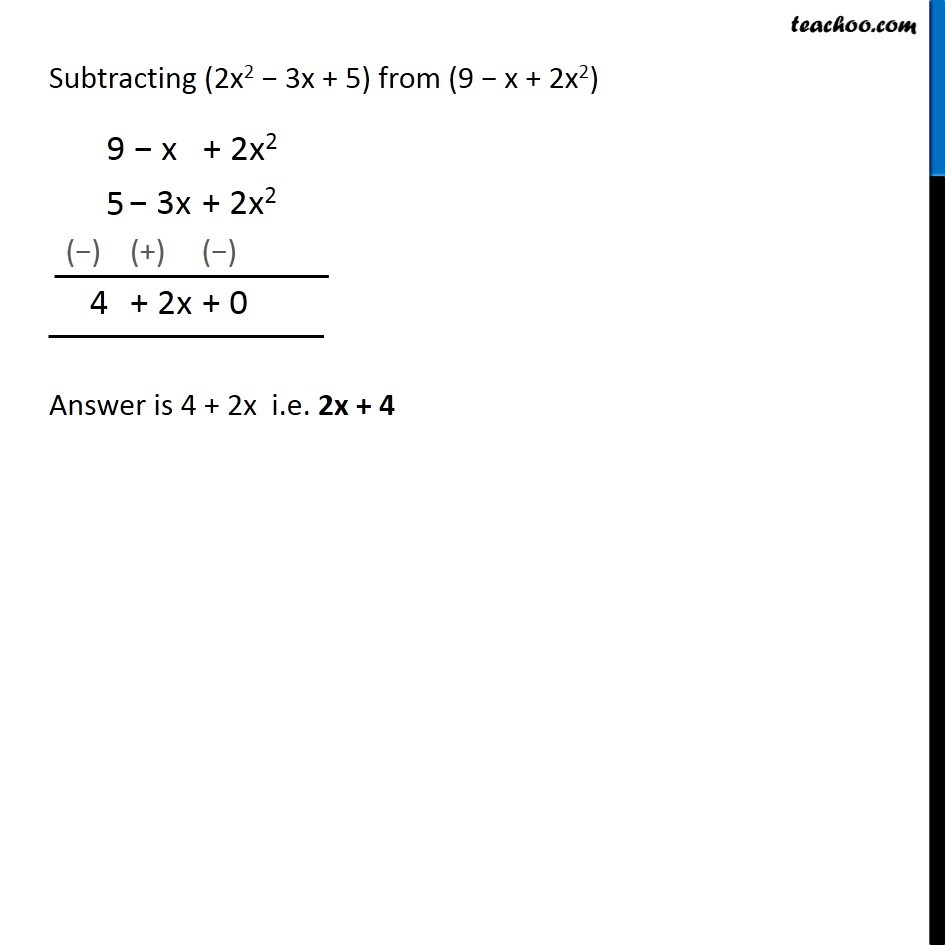Subscribe to our Youtube Channel - https://you.tube/teachoo

1. Chapter 12 Class 7 Algebraic Expressions
2. Serial order wise
3. Ex 12.2

Transcript

Ex 12.2, 6 (a) From the sum of 3x – y + 11 and – y – 11, subtract 3x – y – 11. Sum of (3x − y + 11) and (−y −11) is given by 3x − y + 11 − y − 11 Now, subtracting (3x − y − 11) from (3x − 2y) Answer is −y + 11 Ex 12.2, 6 (b) From the sum of 4 + 3x and 5 – 4x + 2x2, subtract the sum of 3x2 – 5x and –x2 + 2x + 5. Sum of (4 + 3x) and (5 − 4x+ 2x2) is given by Sum of (3x2 − 5x) and (−x2 + 2x + 5) is given by Subtracting (2x2 − 3x + 5) from (9 − x + 2x2) Answer is 4 + 2x i.e. 2x + 4

Ex 12.2